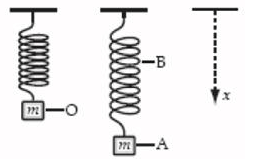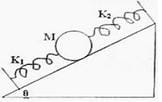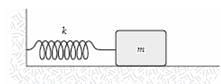NEET  >  Test: Examples of Bodies in Simple Harmonic Motion

# Test: Examples of Bodies in Simple Harmonic Motion

Test Description

## 5 Questions MCQ Test Physics Class 11 | Test: Examples of Bodies in Simple Harmonic Motion

Test: Examples of Bodies in Simple Harmonic Motion for NEET 2023 is part of Physics Class 11 preparation. The Test: Examples of Bodies in Simple Harmonic Motion questions and answers have been prepared according to the NEET exam syllabus.The Test: Examples of Bodies in Simple Harmonic Motion MCQs are made for NEET 2023 Exam. Find important definitions, questions, notes, meanings, examples, exercises, MCQs and online tests for Test: Examples of Bodies in Simple Harmonic Motion below.
Solutions of Test: Examples of Bodies in Simple Harmonic Motion questions in English are available as part of our Physics Class 11 for NEET & Test: Examples of Bodies in Simple Harmonic Motion solutions in Hindi for Physics Class 11 course. Download more important topics, notes, lectures and mock test series for NEET Exam by signing up for free. Attempt Test: Examples of Bodies in Simple Harmonic Motion | 5 questions in 10 minutes | Mock test for NEET preparation | Free important questions MCQ to study Physics Class 11 for NEET Exam | Download free PDF with solutions
 1 Crore+ students have signed up on EduRev. Have you?
Test: Examples of Bodies in Simple Harmonic Motion - Question 1

### A weight of mass m is at rest at O when suspended from a spring, as shown. When it is pulled down and released, it oscillates between positions A and B. Which statement about the system consisting of the spring and the mass is correct?Test: Examples of Bodies in Simple Harmonic Motion - Question 2

### What provides the restoring force for SHM in case of column of mercury in U-tube?

Detailed Solution for Test: Examples of Bodies in Simple Harmonic Motion - Question 2

Weight of mercury provides the restoring force for SHM in case of column of mercury in U-tube.

Test: Examples of Bodies in Simple Harmonic Motion - Question 3

### A sphere of mass M is arranged on a smooth inclined plane of angle Î¸, in between two springs of spring constants K1 and K2 . The springs are joined to rigid supports on the inclined plane and to the sphere (Fig). When the sphere is displaced slightly, it executes simple harmonic motion. What is the period of this motion?Detailed Solution for Test: Examples of Bodies in Simple Harmonic Motion - Question 3

Compression and expansion in both the springs will be same. Hence, F = k1x= k2x. Fn=F + F. Therefore, kn = k1 + k2.
T = 2π [m / kn]½
T = 2π [m / (k1+k2)]½
Hence A is correct.

Test: Examples of Bodies in Simple Harmonic Motion - Question 4

The bob of a pendulum of length, I is pulled aside from its equilibrium position through an angle θ and then released. what will be the speed V of the bob when it pass through its equilibrium condition.

Test: Examples of Bodies in Simple Harmonic Motion - Question 5

A mass m = 2.0 kg is attached to a spring having a force constant k = 290 N/m as in the figure. The mass is displaced from its equilibrium position and released. Its frequency of oscillation (in Hz) is approximately:Detailed Solution for Test: Examples of Bodies in Simple Harmonic Motion - Question 5

We know that T = 2 π√m/k
And f = 1/ T
Thus we get f = 1.9Hz

## Physics Class 11

127 videos|464 docs|210 tests
 Use Code STAYHOME200 and get INR 200 additional OFF Use Coupon Code
Information about Test: Examples of Bodies in Simple Harmonic Motion Page
In this test you can find the Exam questions for Test: Examples of Bodies in Simple Harmonic Motion solved & explained in the simplest way possible. Besides giving Questions and answers for Test: Examples of Bodies in Simple Harmonic Motion , EduRev gives you an ample number of Online tests for practice

## Physics Class 11

127 videos|464 docs|210 tests

### How to Prepare for NEET

Read our guide to prepare for NEET which is created by Toppers & the best Teachers